﻿ Modified Rayleigh Model in the Interpretation of Soil Moisture Data
Hydrology: Current Research

Like us on:

All submissions of the EM system will be redirected to Online Manuscript Submission System. Authors are requested to submit articles directly to Online Manuscript Submission System of respective journal.
• Research Article
• Hydrol Current Res 2012, Vol 3(2): 129
• DOI: 10.4172/2157-7587.1000129

# Modified Rayleigh Model in the Interpretation of Soil Moisture Data

Jitendra Behari* and V. Sharmendra
School of Environmental Sciences, Jawaharlal Nehru University, New Delhi, India
*Corresponding Author: Jitendra Behari, School of Environmental Sciences, Jawaharlal Nehru University, New Delhi, India, Email: [email protected], [email protected]

Received Date: Jan 06, 2012 / Accepted Date: Apr 16, 2012 / Published Date: Apr 22, 2012

### Abstract

Surface soil moisture is important to understand environmental systems and dielectric constant is a convenient physical parameter to describe this. Dielectric Mixing models relate the effective dielectric constant of a heterogeneous medium with the properties of constituent materials. The dielectric properties of the soil is known to have dependence on Soil moisture content along with other factors like texture, frequency, salinity etc. In this investigation, experimental data of Dielectric Constant of various types of soil has been taken at 1.4 GHz and 18 GHz to fit unknown parameters in modified Rayleigh mixing model. Calculation of Effective dielectric constant and emissivity of different types of soil has been carried out and using modified Debye formulation, spread in relaxation and relaxation frequency is also obtained. Effective dielectric constant increases with rise in volumetric Soil Moisture, while the emissivity decreases. Further the emissivity increases with the increase in Roughness parameter. Relaxation Frequency (fr) and spread of relaxation (α) are identified as microscopic parameters to characterize the soil texture along with volumetric moisture. These parameter values decreases with an increase in soil moisture. These data can be obtained in laboratory and field conditions and can be of interest to agricultural scientists.

Keywords: Soil moisture; Dielectric constant; Emissivity; Roughness parameter

#### Abbreviations

α: Spread of Relaxation; ρs: Bulk Density; ρr: Density of Rock; λ: Wave length of the Electric Field; ε: Complex Dielectric Constant of Soil; εa: Dielectric Constant of Air; εbw: Dielectric Constant of Bound Water; ε1: Dielectric Constant; εfw: Dielectric Constant for the Free Water; εeff: Effective Dielectric Constant of the Mixture; εdry soil: Effective Dielectric Constant for Dry Soil; εs: Static Dielectric Constant; esoil: Soil Emissivity; ε: High Frequency Limit of Dielectric Constant; f1: Volume Fraction of the Scatterer; fr: Relaxation Frequency; fbw: Volume Fraction of Bound Water; ffw: Volume Fraction of Free Water; ω: Angular Frequency; τ: Relaxation Time; θ: Angle of Incidence; θ’: Subsurface Incidence Angle; mv: Volumetric Moisture.

#### Introduction

Soil moisture is an environmental parameter and is an interface between the earth surface and the atmosphere. The soil moisture plays an important role in influencing the climate change by altering the surface albedo, soil heat capacity and the heat flux between air and land . Usually the soil temperature and moisture forecasting has been performed using a land surface model (LSM). This approach models heat transfer and moisture flow between the atmosphere and the soil surface. However this demands knowledge of a large number of parameters for different soil types. Some authors [2,3] have adopted a data mining approach for soil moisture prediction: a machine learning approach using a regression free algorithm, based upon current soil state and atmospheric conditions. These authors derived a linear equation to the highly non-linear LSM processes.

Recent advances in remote sensing technology have shown that soil moisture can be measured by a variety of techniques using non ionizing parts of the electromagnetic spectrum. However microwave technology has the potential to operate from a space platform and has proven ability to quantitatively measure soil moisture under a variety of conditions. In microwave remote sensing, the most important parameter is the knowledge of dielectric constant of soils [4-7]. Accurate measurement of the complex dielectric properties of soils are necessary to predict how fast electromagnetic waves will travel through the soil and how quickly these will be attenuated . In pursuance of this several attempts have been undertaken for the measurement of the relative permittivity of soil [9-12] and its theoretical formulation with water mixture (with and without consideration of bound water fraction) at microwave frequencies [13-20,6]. An important parameter in this connection appears to be an explicit consideration of bound water fraction along with the solid texture. Bound water is held in soil by electromolecular forces. It can be imagined that the closer the water layer to the particle, more of it is distorted with respect to free water and ice. This brings about difference in various physical properties of soil .

Soils are complex systems consisting of varying proportions of soil matrix, water and air . Soils are highly heterogeneous in their physical properties and chemical composition and variable in their spatial distribution. To account for this, surface soil moisture quantification models from reflectance data under field conditions have been developed . In this formulation the specific characteristics of soil types are taken into account by considering the ratio of the measured reflectance and that of the reference samples under dry conditions. To interpret the soil moisture data, Whiting et al.  proposed the fitting of an inverse Gaussian model. The idea is to account for the reflectance decrease towards the water absorption band at 2800 nm. This approach is simple and can be worked out in a laboratory set up.

The soil moisture forecast from the physical model thus remains a major challenge. An ideal model formulation would provide for the observed effects of various soil components (particle size distribution and mineralogy) on the complex dielectric behavior of the soil- waterair system as a function of frequency. This would also include soil bulk density, volume fraction of soil water components, salinity of the soil solution and temperature. In pursuance of these a number of mixing formulae (e.g. Wang and Schamugge ) have been attempted, which inadequately describe the soil behavior in the frequency range of interest. A comprehensive account of this is given elsewhere . Many of these formulae are unable to account for the complex behavior of soil medium over a wide of frequency range (1.4 GHz –18.0 GHz).

There is no exact solution for the electromagnetic problem with random parameters and boundaries. Therefore, Mixing theories have, been put in use. The basic requirements for their validity and use are: the spatial variation of the electronic field has to be less than the variation in the structure of the medium. This means that the sizes of the inclusions of the mixture or the correlation distance (in case the medium is describable by continuous dielectric function) has to be considerably smaller than the wave length of the electric field λ. The requirement is that the maximum chord of an inclusion is about λ/2π. For the boundaries with a spatial variability of the order of wavelength, scattering effects are not negligible, and the concept of effective dielectric constant is not valid. The effective dielectric constant is a complex number and includes losses. These are absorption losses and results from the losses of the constituent components.

The dielectric models provide convenient means for predicting soil dielectric behavior for use in microwave emission and scattering calculations, and more importantly theoretical mixing models that depends upon measurable soil parameters only, i.e. demanding no adjustable parameters. Approaches have primarily been focused on the microwave part of the spectrum, because the moisture strongly affects soil dielectric properties and longer wavelengths make a relatively deep penetration into the ground possible . Soil texture affects the microwave sensing of soil moisture in a way that the dielectric constant changes with the relative amounts of sand, silt and clay in the soil. Oh et al.  have developed an empirical model in terms of root mean square height, the wave number and the relative dielectric constant. By using this model with multipolarized radar data the soil moisture content and the surface roughness can be determined.

The aim of the present study is to step towards finding a robust comprehensible and easily applicable model, demanding least number of input parameters for the determination of surface soil moisture which can be applied not only under laboratory, but also under field conditions. This demands an empirical formulation, taking into consideration a four soil components (air, water (bound, free), soil matrix) which predicts variation of dielectric constant with moisture and frequency. This has been incorporated using modified Rayleigh’s formulation.

#### Theoretical Formulation

Raleigh considered scatterers as spherical and in his formulation this is written as: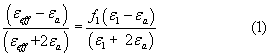εeff is effective dielectric constant of the mixture, f1 is volume fraction of the scatterer and ε1 its dielectric constant and εa dielectric constant of air.

From equation (1), it is obvious that when f1 = 0, εeff = εa, when f1 = 1, εeff = ε1 and this complies with the physical situation.

Equation (1) mentioned above involves the assumptions; (a) particles are spherical in shape, and (b) there is no interaction between the particles (i.e. they behave independently of each other). It can be imagined that soil particles (scatterers) may not be spherical, but of any arbitrary shape. The interaction between soil particles may also be taken into accounts. The water fraction can be split into two: (free water fraction) (X) and bound water (1 –X). Taking these into consideration, equation (1) can be rewritten as: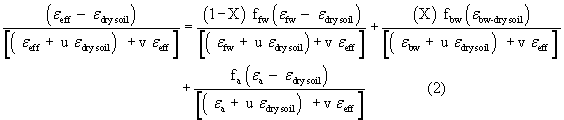Where

εeff = Effective Dielectric Constant, εa = Dielectric constant of air, εdry soil=Effective Dielectric Constant for Dry soil, εfw= Dielectric Constant for the Free Water, fbw= Volume Fraction of Bound water, ffw =Volume Fraction of Free water.

Modified Rayleigh model contains three free parameters u, v and x. v as an indicator of causing polarization because of charge overlap among solid particles. This is for one dimensional scatterer. The value of v may depend on the filling factor and hence could be different for sparse and dense mixtures. For the sparse mixtures the effect of v is small. The particles may have any arbitrary shape for the inclusions, and value of u will vary depending upon its shape and is equal to 2.0 for spherical inclusions, which may also depend on filling factor. Using computed values of u, v and x the dielectric data so obtained are interpreted to describe the soil moisture.

#### Method And Computation

Real and imaginary parts of the dielectric constant of water are best described by Debye’s equation.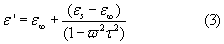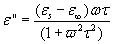Where

ε, Complex Dielectric Constant of the soil; εs, Static Dielectric Constant; ε, High Frequency limit of dielectric constant; ω, Applied Angular Frequency (2πf ); τ, is the relaxation time (time required for the water molecule to align itself along with an applied field).

Various forms of Debye’s equation have been proposed to describe the behavior of water, one of which is the Cole-Cole equation. (Equation 4) .

Dielectric Constant of bound water (εbw) has been calculated from the Cole-Cole Equation , given as: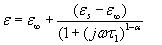Where, τ1= 9.3 x 10-6 s (4)

Where,

Dielectric Constant of air (εa) is taken as 1-j0; Dielectric Constant of dry soil (εdry soil) is taken as 4.7 – j 0.05, Porosity is computed as below: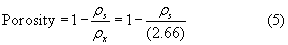Where, ρs = bulk density (gm/cm3); ρr = density of rock (2.66) (gm/ cm3), depending on the mineralogical composition of the parental rock. Volume Fraction of air (fa) is defined as fa = porosity – volumetric moisture Content, while, Volume fraction of bound water (fbw) = volumetric moisture content multiplied by “X”. Volume fraction of free water (fw) = volumetric moisture content multiplied by (1-X).

#### Relaxation Frequency (fr = 1/t) and Spread of Relaxation (a)

Relaxation Frequency (fr) and Spread of Relaxation (α) are, in general, functions of texture and moisture. The complex value (ε = ε’ - jε”) are entered in equation (4) and the corresponding values of spread of relaxation (α) and relaxation frequency (fr) are computed.

A related parameter connected to dielectric constant is the soil emissivity. Fresnel’s equation connects these and is presented below: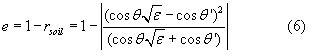Where esoil = soil emissivity, θ = angle of incidence (with the normal), ε = complex dielectric constant of soil and θ’ = subsurface incidence angle.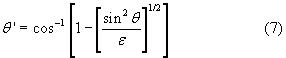The emissivity of ground surface in terms of soil emissivity and roughness parameter (h) is given by

esurface = 1 + (e - 1) exp h (8)

Since soil emissivity depends to a large extent on the complex dielectric constant, which in turn, depends on the volumetric moisture content (mv), one can directly correlate emissivity with mv. At normal incidence, the emissivity of a soil surface increases with increasing degree of surface roughness.

In our model u, v, x are free parameters which are adjusted to achieve a best fit with the experimental ones. To know these unknown parameters, experimental values of dielectric permittivity of various soil samples is taken from Hallikainen results  and computation of effective dielectric constant and emissivity are carried out using modified Rayleigh’s model. It can be seen as an indicator of how the polarization of neighboring inclusions is taken into account in calculating the dipole moment of a single scatterer v for one dimensional scatterer. The dielectric data so obtained are interpreted to characterize soil texture at the microscopic level. The values of u, v and x are computed for different textures ( - Table 1), frequency (1.4 GHz-18 GHz) and volumetric soil moisture (20-30%). The experimental data for soil moisture has been obtained from the experimental work of Hallikainen et al.  in the mentioned soil moisture range. These authors have used waveguide technique at 1.4GHz and 4-6GHz with the free space transmission technique at 4-18 GHz in 2 GHZ increments. The texture composition of the soil for four samples used by them is adopted here. The role of bound and free water is crucial for estimation of dielectric constant and this in turn is related to soil texture. This becomes more important with the increase of volumetric soil moisture. However, for dry soil texture is relatively unimportant for computation of dielectric data.

Sample Number Soil Types Sand Silt Clay
1 Sandy Loam 51.5 35.1 13.4
2 Loam 42.0 49.5 8.5
3 Silt Loam 30.6 55.9 13.5
4 Silt Clay 5.0 47.6 47.4

Table 1: The texture content of different types of Soils.

The regression equation so obtained, can be used for any soil texture within volumetric moisture limit 0% - 40%. We then optimize the values of u, v, and x through the method of iteration to predict known experimental values. In the present study, we have computed the dielectric constant (using Equation 2) of a given soil type at any moisture content and frequency, using regression equations (Equations 9-13). A summary of the computation procedure is shown in following flow chart.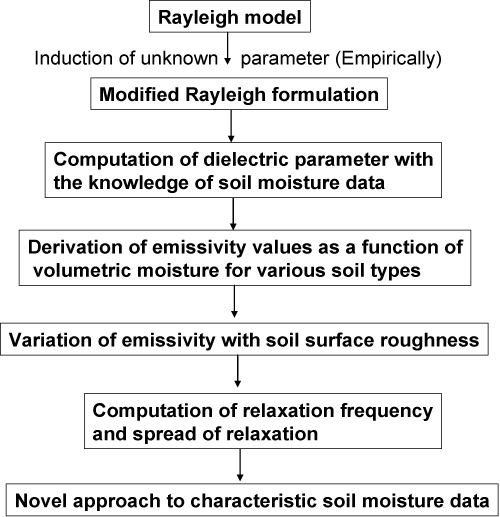#### Results and Discussions

From Figures (1-3), a non linear behavior in dielectric constant, at all the three frequencies is evident for varying soil texture. The degree and mode of variation in dielectric constant would be determined by the texture type and the variation in volumetric soil moisture.

We observe a good agreement with the Hallikainen’s experimental results for computed dielectric data (Figures 1-3). A regression analysis of the dependence of these three parameters on texture and moisture results in the following expressions:

Figure 1: Variation of Dielctric constant with the increase in volumetric soil moisture at 1.4 GHz obtained with the computed values of u, v, and x (equation 2). The texture data are as shown in table 1, where the computations are carried out at descrete points (shown as markers).

Figure 2: Variation of Dielctric constant with the increase in volumetric soil moisture at 5 GHz obtained with the computed values of u, v, and x (equation 2). The texture data are as shown in table 1, where the computations are carried out at discrete points (shown as markers).

Figure 3: Variation of Dielctric constant with the increase in volumetric soil moisture at 18 GHz obtained with the computed values of u, v, and x (equation 2). The texture data are as shown in table 1, where the computations are carried out at discrete points (shown as markers).

X=0.976+ (-0.00999)*Sa (%) + (-0.00949)* Si (%) + (- 0.00869)*Cl (%) + 0.00411* f + (0.00326)* mv (9)

U=0.1530+ (0.0225)*Sa + (0.0223)*Si + (0.0226)*Cl + (0.00316)*f + (0.0164)*mv (10)

V=0.268*Si (%) +0.260*Si (%) +0.261*Cl (%) + (0.0505)*f+0.0117* mv (11)

Where sand (%), silt (%) and clay (%) refer to the soil texture (%) and mv is the volumetric soil moisture. Programs have been developed by using Turbo C compiler to carry out the entire set of computations.

A regression analysis of the dependence of these two parameters (texture and moisture) results in the following expressions:

fr(MHz)=(8.296)*Sa(%) +(11.190)*Si (%) +(5.957)*Cl (%) + (-473.651)*mv+ (-503.56) (12)

α=(0.000629)*Sa(%)+(0.00122)*Si(%)+(0.000768)*Cl(%)+(- 0.0806)*mv+(-0.00907) (13)

Moisture content

Theoretical model accurately predicts an increase of ε` with mv for frequencies under consideration, whereby the values of ε‘for soil samples, are plotted against volumetric moisture content (mv).

In Table 2-4, the dielectric constant of various types of soil at 1.4 GHz, 5 GHz and 18 GHz is shown and a comparison between experimental and calculated results are presented (Δε’ = ε’experimental - ε’calculated). Figures 1-3 shows that an increase in ε’ experimental (experimental) and corresponding ε’computed (theoretical) data at various frequencies and are found to in good agreement. The results have been plotted to show the relationship more explicitly. The relationship between the experimental and calculated values can be represented as ε’experimental = A+B ε‘calculated, where A and B are coefficients for a given soil type at specified locations (Tables 2-4). Since the gap between experimental and theoretical data is small, a linear relationship between the two is envisaged.

Δε' = ε'experimental - ε'calculated , ε'experimental = A+B ε'calculated
1.4 GHz
Modified Rayleigh Mixing Model
Sandy Loam Loam Silt Loam Silt Clay
Δε' (10%) 0.12 0.23 0.63 -0.16
Δε' (20%) 0.13 0.37 0.28 -0.23
Δε' (30%) 0.42 0.43 0.18 -0.37
Δε' (40%) 0.42 1.9 1.22 -1.14
A -0.16 0.08 -0.20 -0.318
B 0.99 0.93 0.96 1.06
R2 0.99 0.98 0.99 0.99
SE 0.13 0.43 0.39 0.20

Table 2: The difference (Δε’) of experimental and calculated dielectric constant at 1.4GHz. A linear relationship has been found at various volumetric moisture.

Δε' = ε'experimental - ε'calculated , ε'experimental = A+B ε'calculated
5 GHz
Modified Rayleigh Mixing Model
Sandy Loam Loam Silt Loam Silt Clay
Δε' (10%) 0.72 0.96 1.03 -0.66
Δε' (20%) -0.43 -0.04 0.12 -1.1
Δε' (30%) 0.59 0.64 0.77 -0.75
Δε' (40%) 1.77 0.45 0.47 -0.53
A -0.08 -0.67 -0.73 0.36
B 0.95 1.01 1.0139 1.02
R2 0.99 0.99 0.99 0.96
SE 0.74 0.40 0.38 0.54

Table 3: The difference (Δε’) of experimental and calculated dielectric constant at 5.0GHz. A linear relationship has been found at various volumetric moisture.

Δε' = ε'experimental - ε'calculated , ε'experimental = A+B ε'calculated
18 GHz
Modified Rayleigh Mixing Model
Sandy Loam Loam Silt Loam Silt Clay
Δε' (10%) 0.26 1.06 1.27 1.13
Δε' (20%) 0.56 0.36 0.47 -0.26
Δε' (30%) 0.55 0.37 0.32 -0.56
Δε' (40%) -1.28 -0.83 -0.2 -1.07
A -0.61 -1.02 -1.01 -0.99
B 1.093 1.11 1.06 1.14
R2 0.994 0.98 0.96 0.97
SE 0.538 0.41 0.42 0.51

Table 4: The difference (Δε’) of experimental and calculated dielectric constant at 18GHz. A linear relationship has been found at various volumetric moisture.

Frequency

Decrease in ε` with increasing frequency (Figure 4) can be attributed to (a) Relative textural `compositions of the soils and b) the dispersion due to the presence of free water.

Figure 4: Dielectric constant decreases as the frequency increases at different volumetric soil moisture. The computations are made at discrete points, (shown by markers).

Emissivity

As moisture content of soil increases, emissivity decreases (Figure 5). This decrease is small for the lower (< 10%) Volumetric Moisture (mv) values, but increases with increasing mv. Also, emissivity is almost independent of Soil Texture for dry soil. (mv=0) but not so for the wet soil. This can be attributed to the increasing presence of free water.

Figure 5: Variation of emissivity of the soil decreases with the volumetric soil moisture at different angles [Equation (6) and (7)]. The computations are made at discrete points (shown by markers).

We have seen that emissivity is lowest at normal incidence (θ =0) (Figure 5). As “θ” increases emissivity also increases. This increase is slower at low “mv” values, but increases at larger values of mv.

At all moisture contents, emissivity increases marginally with increase in surface roughness (Figure 6). The decrease in emissivity with increase in moisture content is appreciable for smooth, but nominal for rough surfaces. When the surface is rough, the scattering of the emitted waves increases. An increase in emissivity can be attributed to an increase in the soil surface area that interfaces with the air and which can transmit more upwelling energy.

Figure 6: Variation of Emisssivity with the roughness parameter at different volumetric soil moisture (equation 15). The computations are made at discrete points (shown by markers).

Figures (1-6) show a trend of variability in parameters due to variations in soil moisture as an input parameter, where the computations are carried out at discrete points.

#### Microscopic Parameter Analysis (fr, Δα)

Relaxation frequency and Spread of Relaxation remains constant for free water. In the case of soil, these two factors are functions of texture and moisture . Relaxation frequency has been calculated from Cole-Cole equation, expressed as a function of texture in the volumetric range under investigation (40%) (Equation 11-13). Relaxation frequency is a microscopic phenomenon and is intimately related with soil moisture. It is clear that any increase in moisture leads to ionic movement and its hindrance leads to a decrease in relaxation frequency (Figure 7 and 8). Variation in physical behavior with the addition of moisture content may be attributed to the qualitative change in the mechanism of soil- water interaction in this textural region and it is suggestive that, knowing the relaxation frequency, one can predict the nature of a particular soil.

Figure 7: Relaxation varies at different soil samples at various volumetric soil moistures. The computations are made at discrete points (shown by markers).

#### Conclusions

An empirical model is adopted to describe the observed dielectric constant of soil water mixtures as a function of frequency. Expressions for u, v and x, relaxation frequency and the spread of relaxation are general in character. The only input parameter needed is relative content of soil (clay, silt and sand) and the desired volumetric soil moisture data at selected points. The results are valid upto volumetric soil moisture level of 40% for the selected values of soil texture. This model can be used for calculation of similar data for other soil types.

A finger printing of soil texture is the relaxation frequency, which can be easily computed. The result is a general mixing formula for the effective macroscopic permittivity of the mixture. Dielectric constant data shows that there is a close agreement between the experiment and theory. Equations (9-13) are having a general character and an estimation of ε’ are ε’` can be carried out for a variety of texture types. An earlier proposed model  is successful in predicting soil moisture below 30%. This method has been subsequently modified to incorporate texture, frequency and salinity dependence .

Our empirical model provides a good description of the dielectric behavior of various soil water mixtures. The main reason for the success of the model is that it takes into account the shape factor and interaction factor of the constituents. These two free parameters are denoted as u and v, respectively, and can be shown that for certain positive values of u and integral values of v, it reduces to several previously know mixing formulae (u =2 holds for spherical scatterers, but, not for arbitrarily shaped scatterers).

For one-dimensional scatterers, interaction factor is negligible (i.e. v =0). However, this is, in general, not true in case of three dimensional scatterers. Also, at low volume fractions of the scatterer phase, v has little effect, but for a high dielectric constant (for example, mixtures containing water) and high volume filing ratios, mixing formulae with different values for v predict considerably different results. A larger value for v giving a larger effective permittivity. The physical significance of the quantity v is to represent different ways of taking into account the effect of neighbouring scatterers. Experimental dielectric data would depend on the filling factor (v) and consequently on particle shape (u). It is concluded that the present model is successful in modeling the complex nature of soil moisture with least number of input parameters.

#### References

Citation: Behari J, Sharmendra V (2012) Modified Rayleigh Model in the Interpretation of Soil Moisture Data. Hydrol Current Res 3: 129. Doi: 10.4172/2157-7587.1000129

Copyright: © 2012 Behari J, et al. This is an open-access article distributed under the terms of the Creative Commons Attribution License, which permits unrestricted use, distribution, and reproduction in any medium, provided the original author and source are credited.

Select your language of interest to view the total content in your interested language

##### Recommended Journals
Viewmore
###### Article Usage
• Total views: 12189
• [From(publication date): 8-2012 - Jul 23, 2019]
• Breakdown by view type
• HTML page views: 8362Can't read the image? click here to refresh